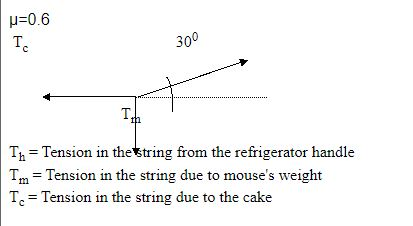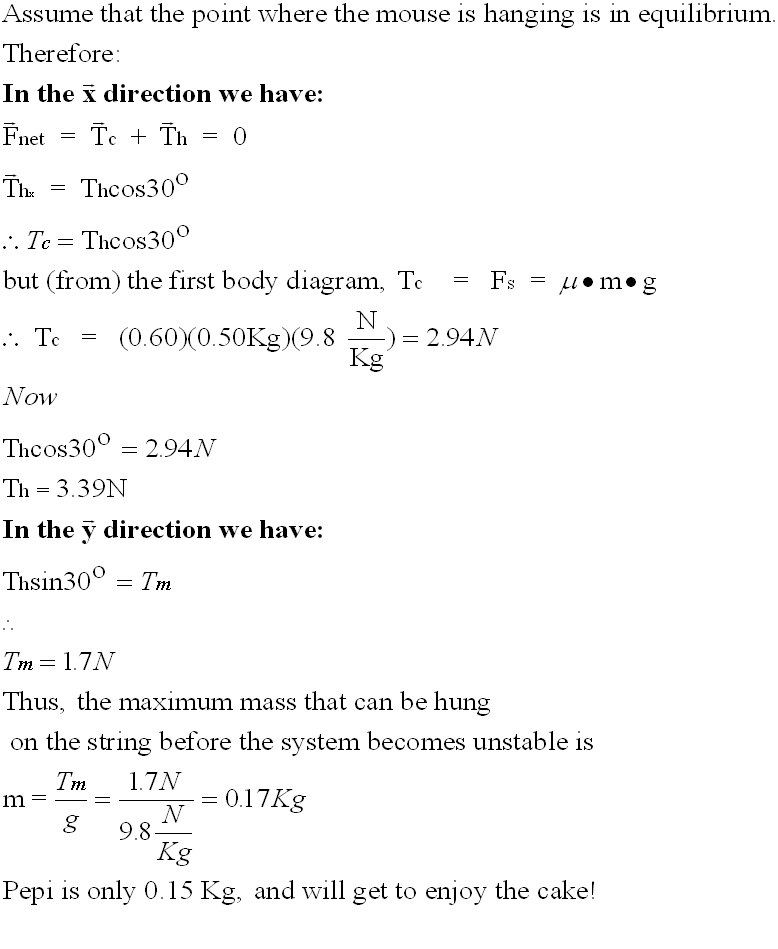### Equilibrium

The word equilibrium derives from an old Latin word meaning to "hold in equal balance" .

In Physics we use the word to mean balanced forces.
• Static
• Dynamic
##### Static Equilibrium
The science of Statics studies how to keep bodies from moving when forces are applied to them.  Generally, we use this science to design and build structures like roads, bridges, buildings, etc.... so that they are stable even under structural stress (such as loads, weights, winds, storms, earthquakes, and so on).

Newton's First Law is the starting point to explain the concept of statics.
Objects at rest will stay at rest.  Object in motion at constant speed will continue to move at constant speed unless acted upon by an external unbalanced force.
Therefore, if the condition of static equilibrium exists, all forces on an object must be balanced.
There are two conditions for static equilibrium to exist.

1. The vector sum of all the forces acting of an object in the x direction must be Zero
2. The vector sum of all the forces acting of an object in the y direction must be Zero

Mathematically, the two conditions above can be written as:Example 1:
Examine the system shown in the diagram below and calculate the tension in both cables.Solution:
i) Find the angles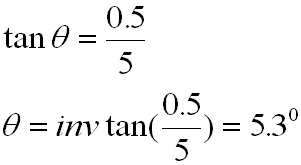ii) Apply the two conditions for static equilibrium - see above notes:Consider the vertical direction: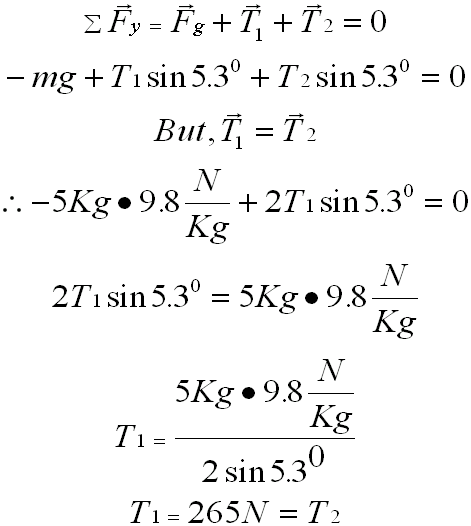Note that in the horizontal direction T1x =  T2x .  This does not give us any additional information about the system and can therefore be disregarded.

Example 2
Problem – Static Equilibrium

A 0.500 kg cake was left overnight on a kitchen counter with a melamine surface having a coefficient of friction of 0.60.
Aware of the presence of mice in the house little Johnny sets up a trap (using his Physics’ knowledge).
He attaches a string from the cake to the refrigerator’s handle and places some sticky paper on the floor below (as shown in the diagram). In the middle of the night, Pepi (a 150 g mouse) climbs the string.
Will Pepi get to eat the cake or will he fall to the floor onto the sticky paper?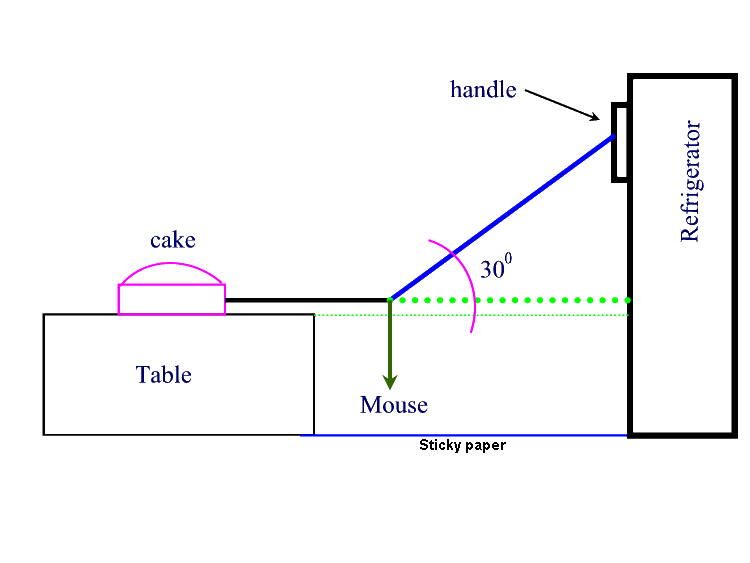Solution to "Pepi & the Cake Problem"

We need to find the maximum mass that the string can handle before either the string breaks or the cake starts to slide along the table top.

There are three free body diagrams to consider.

FBD1:

Cake  Fs   Fs = Force on the String

Tc  Tc = Tension in the string due to the cake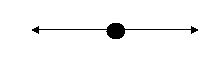Tc   =  Fs

FBD2: